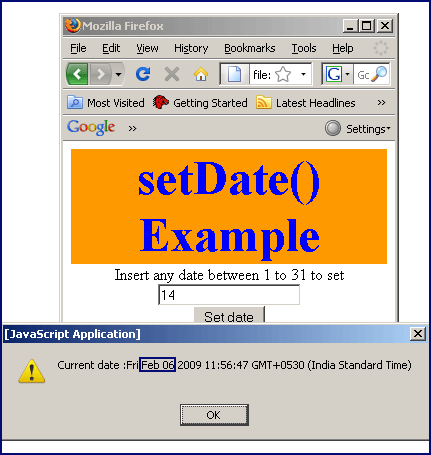Tutorials

JavaScript setDate method

JavaScript setDate() method can be used to set a date value to the date object with an integer value between 1 and 31 that represents day of the current month.

JavaScript setDate() method can be used to set a date value to the date object with an integer value between 1 and 31 that represents day of the current month.

JavaScript setDate method

JavaScript setDate() method can be used to set a date value to the date object with an integer value between 1 and 31 that represents day of the current month. Syntax for using setDate() method is as follows :

Syntax:

 object_date.setDate(dateValue);

Where object_date is the date object which is required to call setDate() method. "dateValue" will hold any integer values between 1 and 31(inclusive) and is required parameter.

Description with example:

In this example we have created a button "Set date". This button when clicked calls the method setDateOfMonth() which is already defined in java script. Here we have taken the current date value into the variable named "dt". In the next line of code we have taken the date value inserted by the user into a variable dateOfMonth. Now we can show current date by using date object "dt" and there after we will call setDate() method to set current date with the value provided by the user into  date reference variable "dt". Here is the small part of code that defines the function :

 function setDateOfMonth() { var dt= new Date(); var dateOfMonth = document.getElementById( 'dateValue').value; if(dateOfMonth >1 && dateOfMonth <31 ){ alert("Current date :" +dt); dt.setDate(dateOfMonth); alert("Date after set :"+dt); }else{ alert("Invalid date"); return false; } }

In the above function we also have applied some validation so that user can enter only valid date to set date. Here is the full example code for setDateExample.html as follows :


setDate() Example
Insert any date between 1 to 31 to set

Output of example :

Suppose we want to set the current date with the date value "14". Insert value 14 into text box and click on the button "Set date".You can see that we have set the date from Feb 06 to Feb 14 which is highlighted in the output.You can also download the full source code from the following link :